• # 概率论与数理统计

## 回顾

### 反函数的导数

设函数 $y=f\left(x\right)$$y=f(x)$ 在区间 ${I}_{x}$$I_x$ 上严格单调、可导，${f}^{\prime }\left(x\right)\ne 0$$f'(x)\neq0$，则它的反函数 $x={f}^{-1}\left(y\right)$$x=f^{-1}(y)$ 在对应的区间 ${I}_{y}=\left\{y|y=f\left(x\right),x\in {I}_{x}\right\}$$I_y=\{y|y=f(x),x\in I_x\}$ 上也严格单调、可导，并且

${{\left({f}^{-1}\left(y\right)\right)}^{\prime }|}_{y=f\left(x\right)}=\frac{1}{{f}^{\prime }\left(x\right)},$

亦即

$\frac{\mathrm{d}x}{\mathrm{d}y}=\frac{1}{\frac{\mathrm{d}y}{\mathrm{d}x}}.$

### 变限积分函数的可微性

• 逐点可导：若函数 $f\left(x\right)$$f(x)$$\left[a,b\right]$$[a,b]$ 上可积，在点 ${x}_{0}\in \left(a,b\right)$$x_0\in(a,b)$ 处连续，则函数

在点 ${x}_{0}$$x_0$ 处可导，且 ${F}^{\prime }\left({x}_{0}\right)=f\left({x}_{0}\right)$$F'(x_0)=f(x_0)$. 若 $f\left(x\right)$$f(x)$$x=a$$x=a$ 点右连续，则 ${F}_{+}^{\prime }\left(a\right)=f\left(a\right)$$F'_+(a)=f(a)$；若 $f\left(x\right)$$f(x)$$x=b$$x=b$ 点左连续，则 ${F}_{-}^{\prime }\left(b\right)=f\left(b\right)$$F'_-(b)=f(b)$.

• 复合函数求导：设函数 $f\left(x\right)$$f(x)$ 在区间 $\left[a,b\right]$$[a,b]$ 上连续，$u\left(x\right),v\left(x\right)$$u(x),v(x)$$\left(\alpha ,\beta \right)$$(\alpha,\beta)$ 内可微，且当 $x\in \left(\alpha ,\beta \right)$$x\in(\alpha,\beta)$ 时，$u\left(x\right),v\left(x\right)\in \left[a,b\right]$$u(x),v(x)\in[a,b]$，则函数

$\left(\alpha ,\beta \right)$$(\alpha,\beta)$ 内可微，且

${\psi }^{\prime }\left(x\right)=f\left(u\left(x\right)\right){u}^{\prime }\left(x\right)-f\left(v\left(x\right)\right){v}^{\prime }\left(x\right).$

### 含参变量积分

#### 含参变量常义积分

设二元函数 $f\left(x,u\right)$$f(x,u)$ 在有界闭区域 $D:a⩽x⩽b,\alpha ⩽u⩽\beta$$D:a\leqslant x\leqslant b,\alpha\leqslant u\leqslant\beta$ 上连续，称积分

为含参变量常义积分，其中 $u$$u$ 称为参变量.

若积分限也依赖于参变量，有变限含参常义积分

##### 含参变量常义积分的可微性
• 如果函数 $f\left(x,u\right)$$f(x,u)$ 在区域 $D$$D$ 上连续，且在 $D$$D$ 上对参变量 $u$$u$ 有连续的偏导数，则函数 $\phi \left(u\right)$$\varphi(u)$$\left[\alpha ,\beta \right]$$[\alpha,\beta]$ 上可导，并且

即可以交换求导运算与积分运算的顺序，或称在积分号下求导；

• 如果函数 $f\left(x,u\right)$$f(x,u)$ 在区域 $D$$D$ 上连续，且在 $D$$D$ 上对变量 $u$$u$ 有连续的偏导数，函数 $a\left(u\right)$$a(u)$$b\left(u\right)$$b(u)$$\left[\alpha ,\beta \right]$$[\alpha,\beta]$ 上都可导，并且 $a⩽a\left(u\right),b\left(u\right)⩽b$$a\leqslant a(u),b(u)\leqslant b$，则函数 $\psi \left(u\right)$$\psi(u)$$\left[\alpha ,\beta \right]$$[\alpha,\beta]$ 上可导，并且

#### 含参变量积分的应用

##### 几个重要的广义积分
• 狄利克雷（Dirichlet）积分

• 概率积分

• 拉普拉斯（Laplace）积分

• 菲涅耳（Fresnel）积分

##### 欧拉（Euler）积分
• $\mathrm{\Gamma }$$\Gamma$ 函数

• 定义：

• 连续性：$\mathrm{\Gamma }$$\Gamma$ 函数在区间 $\left(0,+\mathrm{\infty }\right)$$(0,+\infty)$ 上连续.

• 递推公式：

$x>0$$x>0$ 时，有 $\mathrm{\Gamma }\left(x+1\right)=x\mathrm{\Gamma }\left(x\right)$$\Gamma(x+1)=x\Gamma(x)$. 特别地，

$\begin{array}{c}\mathrm{\Gamma }\left(1\right)=1,\phantom{\rule{1em}{0ex}}\mathrm{\Gamma }\left(n+1\right)=n!,\\ \mathrm{\Gamma }\left(\frac{1}{2}\right)=\sqrt{\pi },\phantom{\rule{1em}{0ex}}\mathrm{\Gamma }\left(n+\frac{1}{2}\right)=\frac{\left(2n-1\right)!!}{{2}^{n}}\sqrt{\pi }.\end{array}$

• 余元公式：

• $\mathrm{B}$$\Beta$ 函数

• 定义：

称为 $\mathrm{B}$$\Beta$ 函数. 其无穷积分表示为

• 连续性：$\mathrm{B}$$\Beta$ 函数在第一象限 $x,y>0$$x,y>0$ 内连续.

• 递推公式：

$\begin{array}{c}\mathrm{B}\left(x+1,y\right)=\frac{x}{x+y}\mathrm{B}\left(x,y\right),\\ \mathrm{B}\left(x,y+1\right)=\frac{y}{x+y}\mathrm{B}\left(x,y\right),\\ \mathrm{B}\left(x+1,y+1\right)=\frac{xy}{\left(x+y\right)\left(x+y+1\right)}\mathrm{B}\left(x,y\right).\end{array}$
• 勒让德（Legendre）加倍公式：

• $\mathrm{\Gamma }$$\Gamma$ 函数与 $\mathrm{B}$$\Beta$ 函数的关系

特别地，有

$\mathrm{B}$$\Beta$ 函数的对称性：$\Beta(x,y)=\Beta(y,x)\ (x,y>0).$

## 第一章 事件及其概率### 1.1 概率论简史

一些基本概念：

• 概率（probability），又称或然率、几率，是表示某个事件出现的可能性大小的一种数量指标，介于 $0$$0$$1$$1$ 之间.

• 赌博问题中的赢率（odds）.

### 1.2 随机试验和随机事件

#### 基本概念

定义1.1 随机试验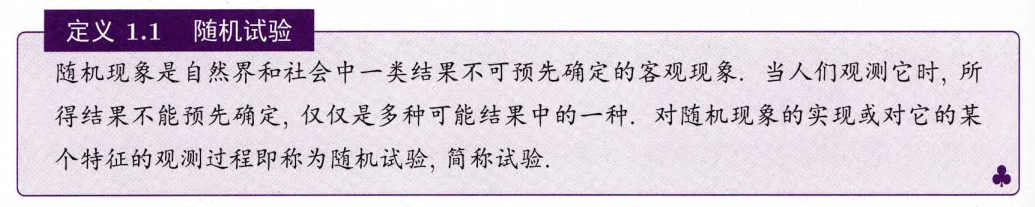定义1.2 样本空间与事件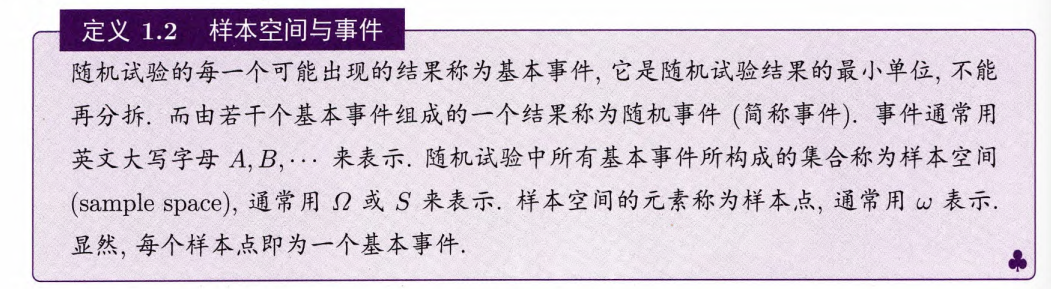• 随机事件（简称 事件），用英文大写字母 $A,\ B,\ \cdots$ 表示；

• 样本空间（sample space）：随机试验中所有基本事件构成的集合，用 $\Omega$$\varOmega$$S$$S$ 表示；

• 样本点：样本空间的元素，即基本事件，用 $\omega$$\omega$ 表示.

一个随机试验的样本空间 $\Omega$$\varOmega$ 是由该试验所有可能结果所组成的集合.

根据样本空间 $\Omega$$\varOmega$ 的大小，可以将其分为三类：

• 有限样本空间（仅含有有限个样本点）；

• 可数无穷样本空间（含有无穷且可数个样本点）；

• 不可数样本空间（含有无穷且不可数个样本点）.

#### 事件的运算

对事件 $A$$A$，如果随机试验的结果恰好出现在 $A$$A$ 中，那么我们就称事件 $A$$A$ 在此次随机试验下发生，简称事件 $A$$A$ 发生.

定义1.3 必然事件和不可能事件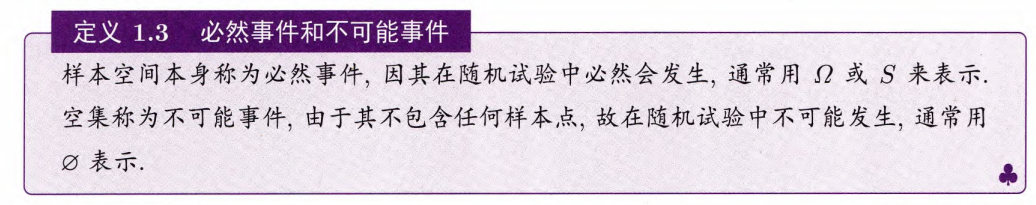习惯上，将必然事件发生的概率设置为 $1$$1$，将不可能事件发生的概率设置为 $0$$0$.

但发生概率为 $1$$1$ 的事件未必是必然事件，发生概率为 $0$$0$ 的时间未必是不可能事件.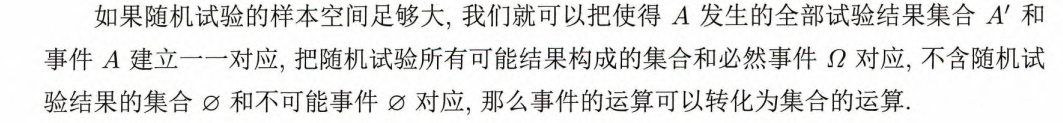定义事件运算中的几个基本概念.

定义1.4 事件的和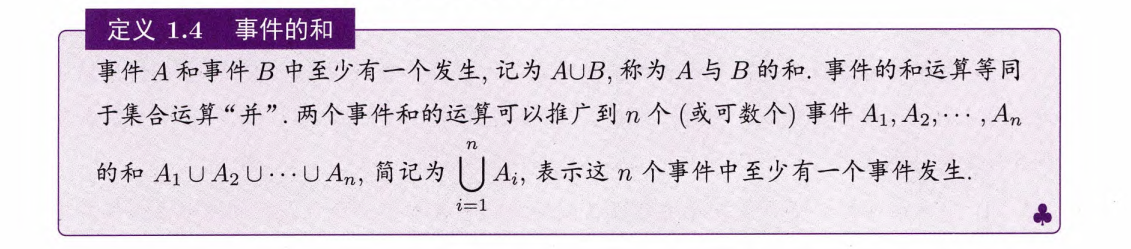事件 $A$$A$ 和事件 $B$$B$ 中至少有一个发生，称为 $A$$A$$B$$B$ 的和，记为 $A\cup B$$A\cup B$.

下面的维恩图（Venn diagram）中阴影部分表示了 $A\cup B$$A \cup B$.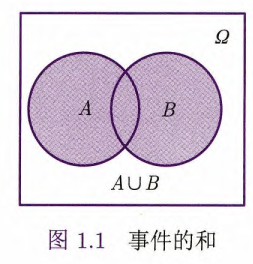定义1.5 事件的差事件 $A$$A$ 发生而事件 $B$$B$ 不发生，称为 $A$$A$$B$$B$ 的差，记为 $A-B$$A-B$$A\stackrel{―}{B}$$A\overline{B}$.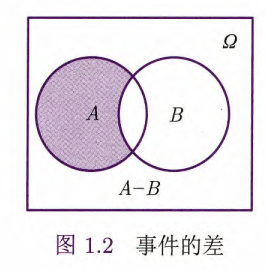定义1.6 事件的积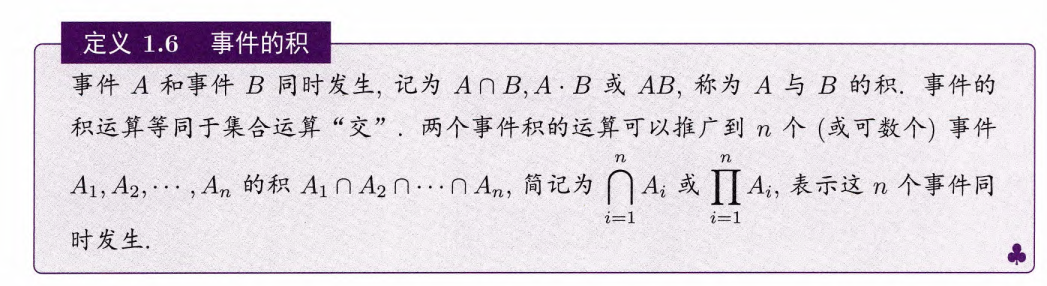事件 $A$$A$ 和事件 $B$$B$ 同时发生，称为 $A$$A$$B$$B$ 的积，记为 $A\cap B$$A\cap B$$A\cdot B$$A\cdot B$$AB$$AB$.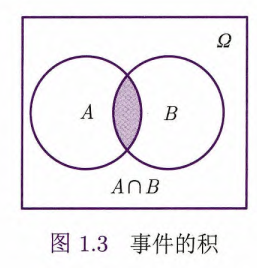定义1.7 不相容事件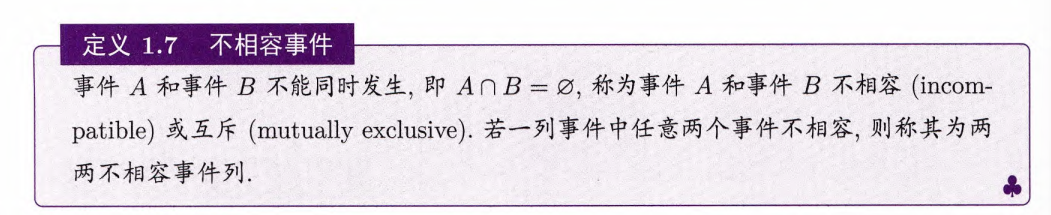事件 $A$$A$ 和事件 $B$$B$ 不能同时发生（即 $A\cap B=\varnothing$$A\cap B=\varnothing$），称为事件 $A$$A$ 和事件 $B$$B$ 不相容（incompatible）或互斥（mutually exclusive）.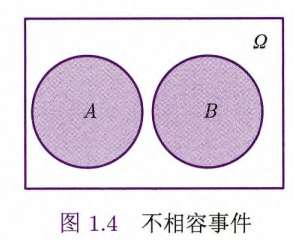特别，当事件两两不相容时，可以把“并”运算符号改写为通常的加号.

定义1.8 对立事件$\{事件\ A\ 不发生\}$ 这一事件称为 $A$$A$ 的对立事件（或余事件），记为 $\stackrel{―}{A}$$\overline{A}$${A}^{c}$$A^c$.

事件运算的公式

• $A\cup A=A$$A\cup A=A$, $A\cap A=A$$A\cap A=A$

• $A\cup BC=\left(A\cup B\right)\left(A\cup C\right)$$A\cup BC=(A\cup B)(A\cup C)$

• $A\cap \left(B\cup C\right)=AB\cup AC$$A\cap(B\cup C)=AB\cup AC$

• $(A\cup B)(C\cup D)=AC\cup BC\ \cup AD\ \cup BD$

• 德摩根（A. De Morgan）对偶法则

• ${\left(\bigcup _{k=1}^{n}{A}_{k}\right)}^{c}=\bigcap _{k=1}^{n}{A}_{k}^{c}$$\displaystyle{\left(\bigcup_{k=1}^nA_k\right)^c=\bigcap_{k=1}^nA_k^c}$

• ${\left(\bigcap _{k=1}^{n}{A}_{k}\right)}^{c}=\bigcup _{k=1}^{n}{A}_{k}^{c}$$\displaystyle{\left(\bigcap_{k=1}^nA_k\right)^c=\bigcup_{k=1}^nA_k^c}$.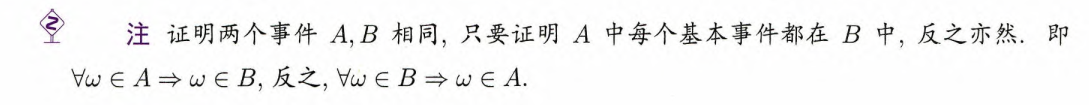证明两个事件 $A$$A$$B$$B$ 相同：$\mathrm{\forall }\omega \in A\phantom{\rule{0.278em}{0ex}}⟹\phantom{\rule{0.278em}{0ex}}\omega \in B$$\forall\omega\in A\implies\omega\in B$$\mathrm{\forall }\omega \in B\phantom{\rule{0.278em}{0ex}}⟹\phantom{\rule{0.278em}{0ex}}\omega \in A$$\forall\omega\in B\implies \omega\in A$.

### 1.3 概率的定义和性质

定义1.9 概率的直观定义概率是事件的函数，也可以视为是集合的函数. 设 $A$$A$ 为一个事件，用 $P\left(A\right)$$P(A)$ 表示事件 $A$$A$ 发生的概率，则由概率定义，

• $0⩽P\left(A\right)⩽1$$0\leqslant P(A)\leqslant1$；、

• $P\left(\Omega \right)=1$$P(\varOmega)=1$

• $P\left(\varnothing \right)=0$$P(\varnothing)=0$.

#### 1.3.1 古典概型

有限性等可能性下定义概率的模型称为古典概型.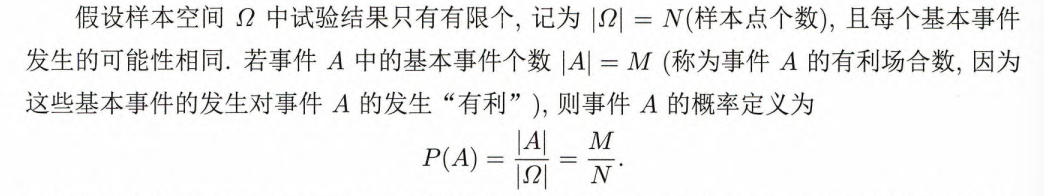一般涉及排列、组合的知识，以及事件的运算. 常用的排列、组合知识归纳如下.

##### 1. 计数原理

加法原理乘法原理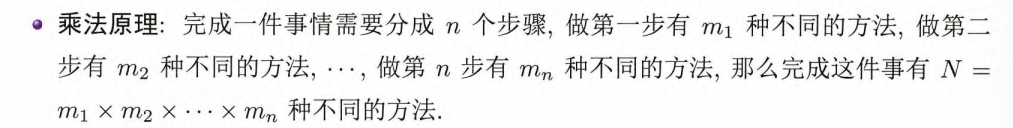结论：

• $n$$n$ 个不同的元素中，有放回地取出 $r$$r$ 个元素组成的可重复排列的不同方式有 ${n}^{r}$$n^r$ 种；

• $n$$n$ 个不同的元素中，不放回地取出 $r$$r$ 个元素组成的不重复排列的不同方式有

${\mathrm{A}}_{n}^{r}=n\left(n-1\right)\cdots \left(n-r+1\right)={\mathrm{P}}_{n}^{r}$

种，称为选排列. 特别，当 $r=n$$r=n$ 时，称为全排列；

• $n$$n$ 个不同的元素中，不放回地取 $r$$r$ 个元素组成的组合，不同方式个数为

${\mathrm{C}}_{n}^{r}=\frac{n\left(n-1\right)\cdots \left(n-r+1\right)}{r!}=\frac{n!}{r!\left(n-r\right)!}=\left(\genfrac{}{}{0}{}{n}{r}\right)$
• $n$$n$ 个不同的元素中，有放回地取 $r$$r$ 个元素组成的组合（不考虑顺序），不同方式个数为

${\mathrm{C}}_{n+r-1}^{r}=\left(\genfrac{}{}{0}{}{n+r-1}{r}\right)$

这个数称为重复组合数 ${\mathrm{H}}_{n}^{r}$$\mathrm{H}_n^r$.

##### 2. 盒子模型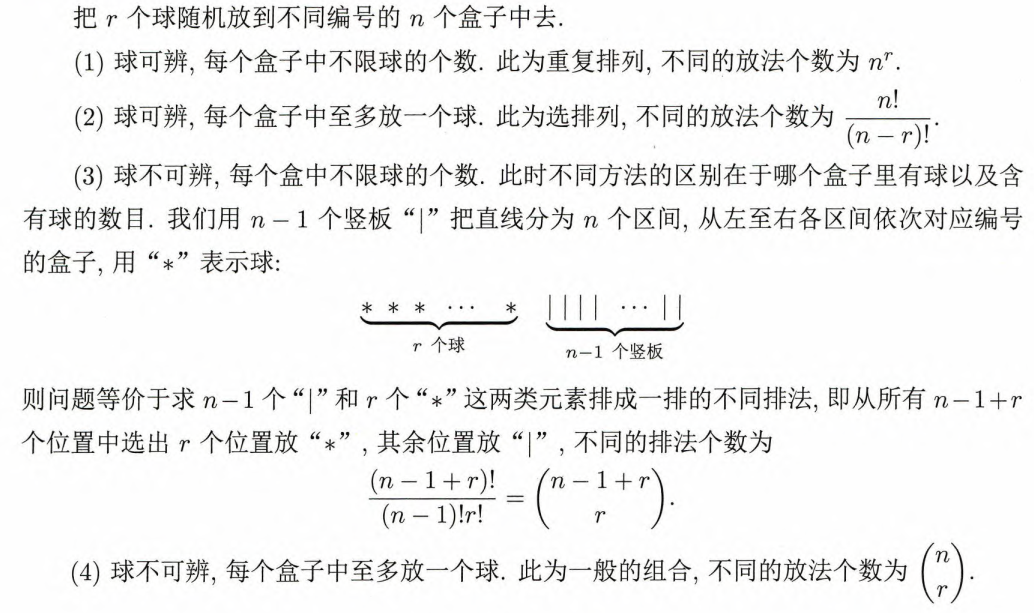结论：

• 球可辨，每个盒子中不限球的个数. 此为重复排列，不同的放法个数为 ${n}^{r}$$n^r$

• 球可辨，每个盒子中至多放一个球，此为选排列，不同的放法个数为

${\mathrm{A}}_{n}^{r}=n\left(n-1\right)\cdots \left(n-r+1\right)=\frac{n!}{\left(n-r\right)!}$
• 球不可辨，每个盒中不限球的个数. 隔板法（$\left(n-1\right)$$(n-1)$ 个隔板，$r$$r$ 个球，共 $\left(n+r-1\right)$$(n+r-1)$ 个位置中放 $r$$r$ 个球，其余 $\left(n-1\right)$$(n-1)$ 个位置中放隔板），则不同的排法个数为

• 球不可辨，每个盒子中至多放一个球. 此为一般的组合，不同的放法个数为

$\left(\genfrac{}{}{0}{}{n}{r}\right)={\mathrm{C}}_{n}^{r}$
##### 3. 多组组合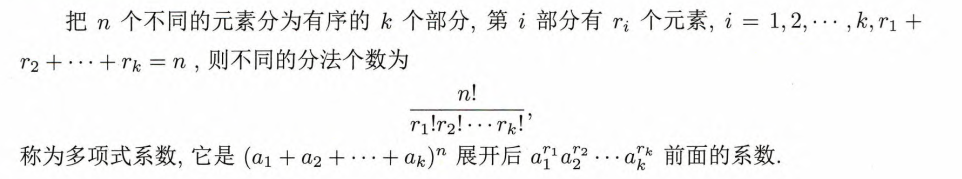##### 4. 不尽相异元素的排列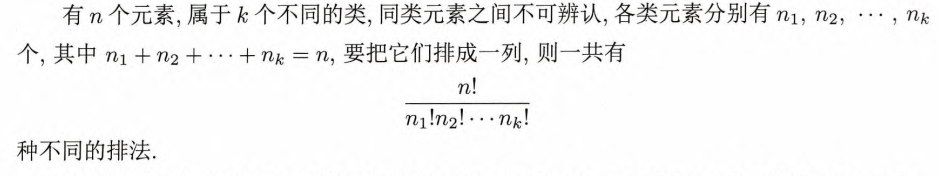#### 1.3.2 概率的统计定义

几何概型：对古典概型去掉有限性、保留基本事件的等可能性.

几何概型相当于把样本空间视为一块质量为 $1$$1$ 的均匀木块，事件 $A$$A$ 视为木块中的某部分，则 $P\left(A\right)$$P(A)$ 就是该部分的质量.

（去掉等可能性，保留有限性，从另一个角度定义概率）

定义1.10 概率的统计定义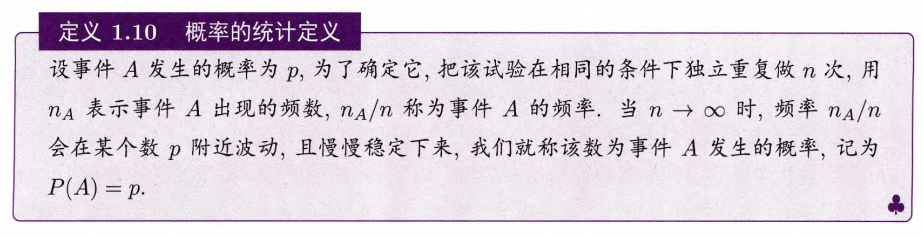意义：

• 提供了一种估计概率的方法（如：得出 $\pi$$\pi$ 的近似值，破译密码）；

• 提供了理论是否正确的标准（如：验证硬币均匀性）.

#### 1.3.3 主观概率的定义

人们常常用一个数字去估计某些概率的大小，而心目中并不把它与频率相连，这种概率称为主观概率.

定义1.11 主观概率定义作用：

• 管理科学（经济投资决策）；

• 数据分析，尤其是人工智能的算法（贝叶斯（T. Bayes）学派，与传统的统计学派即频率学派区别）

研究主观概率，以这种观点来处理统计问题，有着非常重要的现实意义.

#### 1.3.4 概率的公理化定义

定义1.12 概率的公理化定义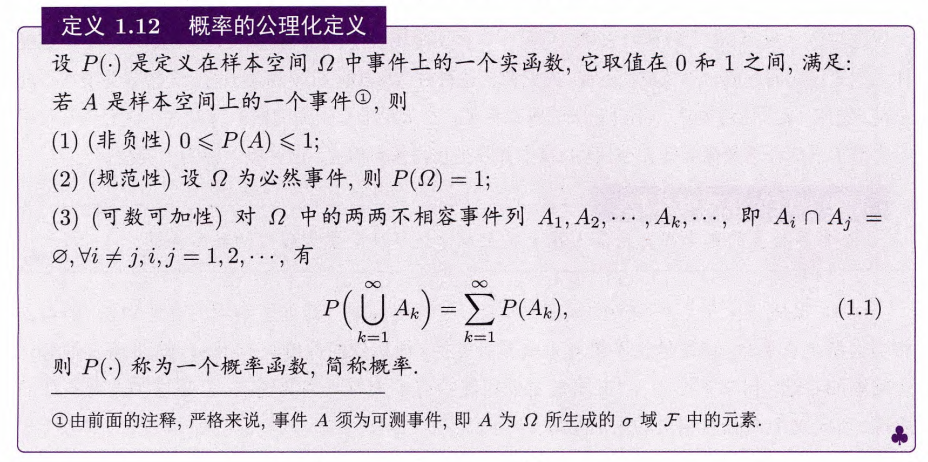由概率的公理化定义得到概率的一些性质. 以下讨论的事件均为同一样本空间 $\Omega$$\varOmega$ 中的可测事件.

• $P\left(\varnothing \right)=0;$$P(\varnothing)=0;$

• （有限可加性）若 ${A}_{k},k=1,2,\cdots ,n$$A_k,k=1,2,\cdots,n$ 两两不相容，则

$P\left(\sum _{k=1}^{n}{A}_{k}\right)=\sum _{k=1}^{n}P\left({A}_{k}\right);$
• （可减性）若 $A\subset B$$A\subset B$，则 $P\left(B-A\right)=P\left(B\right)-P\left(A\right)$$P(B-A)=P(B)-P(A)$;

• （单调性）若 $A\subset B$$A\subset B$，则 $P\left(A\right)⩽P\left(B\right)$$P(A)\leqslant P(B)$;

• $P\left(\stackrel{―}{A}\right)=1-P\left(A\right)$$P(\overline A)=1-P(A)$;

• （加法原理/容斥原理（inclusion-exclusion principle））对任意的事件 ${A}_{1},{A}_{2},\cdots ,{A}_{n}$$A_1,A_2,\cdots,A_n$，有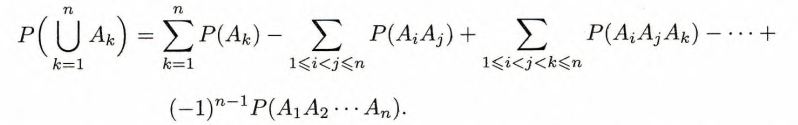例：

$\begin{array}{rl}P\left(A\cup B\cup C\right)& =P\left(A\right)+P\left(B\right)+P\left(C\right)\\ & -P\left(AB\right)-P\left(AC\right)-P\left(BC\right)\\ & +P\left(ABC\right)\end{array}$
• （次可加性）对任意的事件列 ${A}_{1},{A}_{2},\cdots ,{A}_{n},\cdots$$A_1,A_2,\cdots,A_n,\cdots$，有

$P\left(\bigcup _{n=1}^{\mathrm{\infty }}{A}_{n}\right)⩽\sum _{n=1}^{\mathrm{\infty }}P\left({A}_{n}\right);$
• *（下连续性） 若事件列满足 ${A}_{n}\subset {A}_{n+1},n=1,2,\cdots$$A_n\subset A_{n+1},n=1,2,\cdots$，则

$P\left(\bigcup _{n=1}^{\mathrm{\infty }}{A}_{n}\right)=\underset{n\to \mathrm{\infty }}{lim}P\left({A}_{n}\right);$
• *（上连续性） 若事件列满足 ${A}_{n}\supset {A}_{n+1},n=1,2,\cdots$$A_n\supset A_{n+1},n=1,2,\cdots$，则

$P\left(\bigcap _{n=1}^{\mathrm{\infty }}{A}_{n}\right)=\underset{n\to \mathrm{\infty }}{lim}P\left({A}_{n}\right);$

### 1.4 条件概率

#### 1.4.1 条件概率的定义

条件概率，指在试验中在附加一定条件下，感兴趣事件发生的概率，其形式总可归结为“事件 $B$$B$ 发生的条件下事件 $A$$A$ 发生”. 附加的条件一般就是某种信息.

定义1.13 条件概率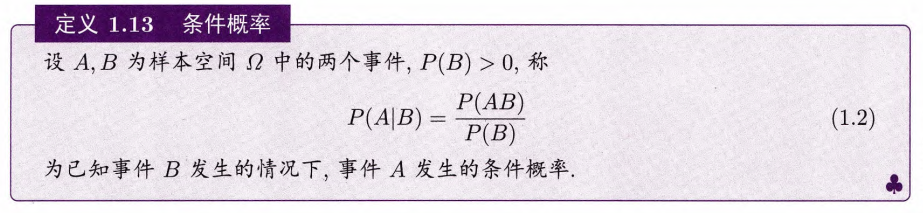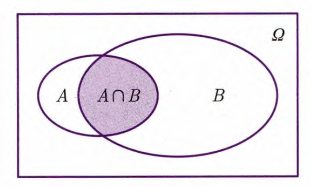某部分的概率就是该部分面积与总面积的比值，图中总面积（$\Omega$$\varOmega$ 的面积）为一个单位. 现在知道 $B$$B$ 发生了，只考虑 $B$$B$ 而不考虑 $\stackrel{―}{B}$$\overline B$，则 $P\left(A|B\right)$$P(A|B)$ 就是 $A$$A$$B$$B$ 中的面积 $P\left(AB\right)$$P(AB)$$B$$B$ 的面积 $P\left(B\right)$$P(B)$ 的比值，即

$P\left(A|B\right)=\frac{P\left(AB\right)}{P\left(B\right)}.$

定理1.1 乘法公式• $P\left(AB\right)=P\left(A\right)P\left(B|A\right)$$P(AB)=P(A)P(B|A)$

• $P\left({A}_{1}{A}_{2}\cdots {A}_{n-1}\right)>0$$P(A_1A_2\cdots A_{n-1})>0$，则

$P\left({A}_{1}{A}_{2}\cdots {A}_{n}\right)=P\left({A}_{1}\right)P\left({A}_{2}|{A}_{1}\right)\cdots P\left({A}_{n}|{A}_{1}{A}_{2}\cdots {A}_{n-1}\right)$

（不依赖脚标顺序）.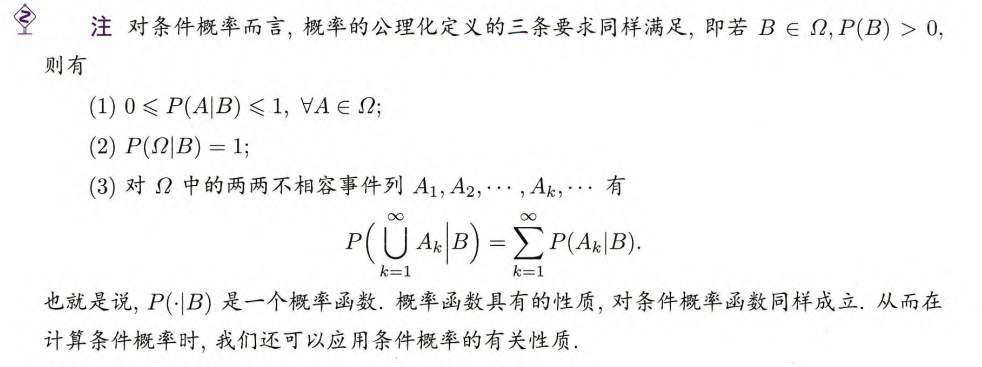#### 1.4.2 全概率公式

定义1.14 完备事件群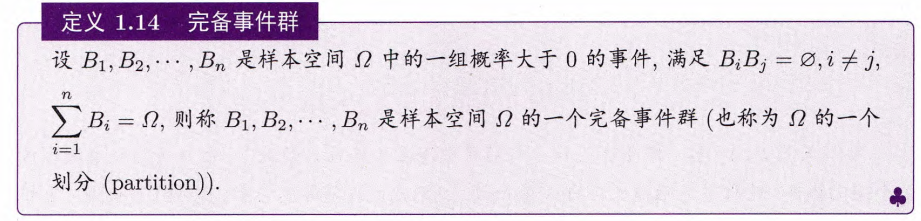${B}_{1},{B}_{2},\cdots ,{B}_{n}$$B_1,B_2,\cdots,B_n$ 是样本空间 $\Omega$$\varOmega$ 中的一组概率大于 $0$$0$ 的事件，满足

• ${B}_{i}{B}_{j}\ne \varnothing ,i\ne j$$B_iB_j\neq\varnothing,i\neq j$

• $\sum _{i=1}^{n}{B}_{i}=\Omega$$\displaystyle{\sum_{i=1}^nB_i=\varOmega}$

则称 ${B}_{1},{B}_{2},\cdots ,{B}_{n}$$B_1,B_2,\cdots,B_n$ 是样本空间 $\Omega$$\varOmega$ 的一个完备事件群（划分（partition））.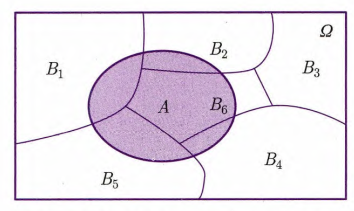定理1.2 全概率公式（law of total probability）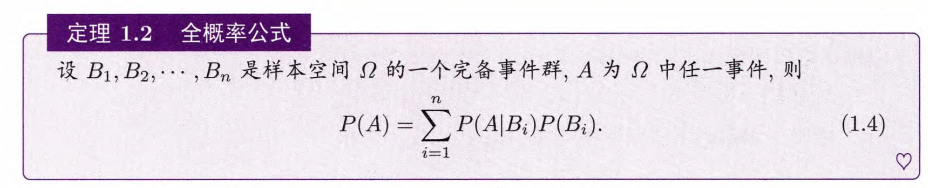${B}_{1},{B}_{2},\cdots ,{B}_{n}$$B_1,B_2,\cdots,B_n$ 是样本空间 $\Omega$$\varOmega$ 的一个划分，$A$$A$$\Omega$$\varOmega$ 中任一事件，则

$P\left(A\right)=\sum _{i=1}^{n}P\left(A|{B}_{i}\right)P\left({B}_{i}\right).$

#### 1.4.3 贝叶斯公式

定理1.3 贝叶斯公式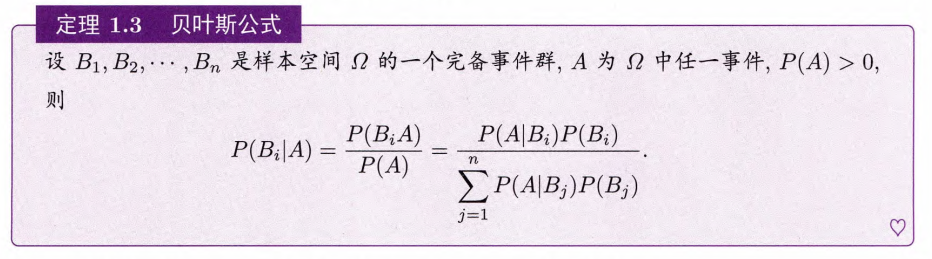${B}_{1},{B}_{2},\cdots ,{B}_{n}$$B_1,B_2,\cdots,B_n$ 是样本空间 $\Omega$$\varOmega$ 的一个划分，$A$$A$$\Omega$$\varOmega$ 中任一事件，$P\left(A\right)>0$$P(A)>0$，则

$P\left({B}_{i}|A\right)=\frac{P\left({B}_{i}A\right)}{P\left(A\right)}=\frac{P\left(A|{B}_{i}\right)P\left({B}_{i}\right)}{\sum _{j=1}^{n}P\left(A|{B}_{j}\right)P\left({B}_{j}\right)}.$

特别，以 $B$$B$$\stackrel{―}{B}$$\overline B$ 构成划分，则

$P\left(B|A\right)=\frac{P\left(A|B\right)P\left(B\right)}{P\left(A|B\right)P\left(B\right)+P\left(A|\stackrel{―}{B}\right)P\left(\stackrel{―}{B}\right)}.$

如果把条件视为“原因”，事件 $A$$A$ 视为“结果”，那么贝叶斯公式反映了因果关系互换之间的概率关系（逆概率（inverse probability））.

### 1.5 独立性

#### 相互独立

定义1.15 两个事件相互独立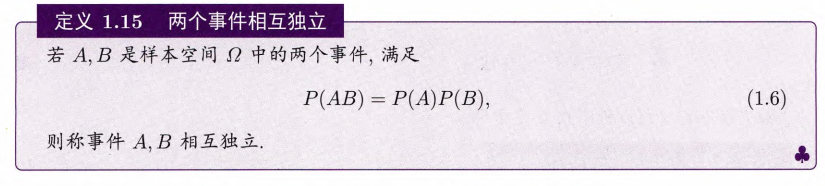如果事件 $A$$A$ 和事件 $B$$B$ 的发生互不影响，那么两事件是独立的.

推论1.1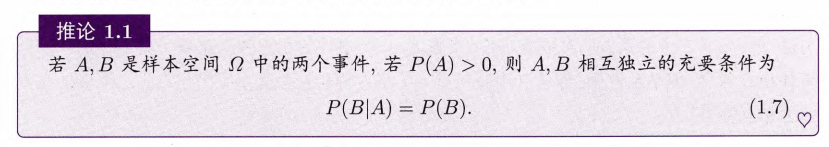两个事件 $A$$A$$B$$B$ 相互独立，实质是一个事件发生的概率与另外一个事件是否发生没有关系，但这并不意味着事件 $A$$A$$B$$B$ 本身完全无关.

定理1.4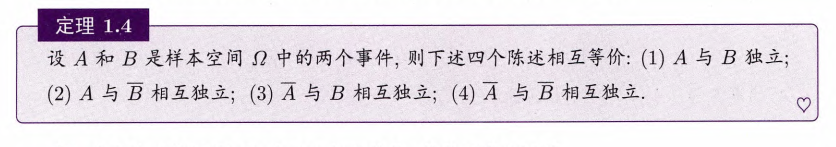$A$$A$$B$$B$ 是样本空间 $\Omega$$\varOmega$ 中的两个事件，则下述四个陈述相互等价：

1. $A$$A$$B$$B$ 独立；

2. $A$$A$$\stackrel{―}{B}$$\overline B$ 相互独立；

3. $\stackrel{―}{A}$$\overline A$$B$$B$ 相互独立；

4. $\stackrel{―}{A}$$\overline A$$\stackrel{―}{B}$$\overline B$ 相互独立.

定义1.16 $n$$n$ 个事件相互独立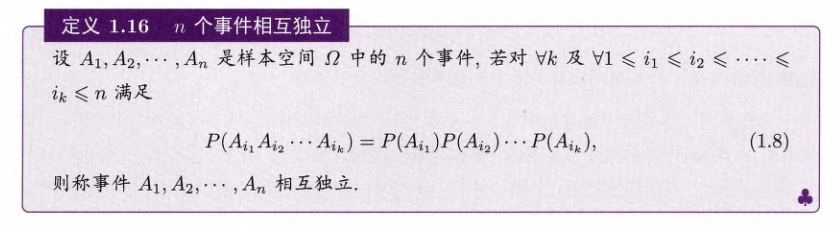$n$$n$ 个事件的相互独立蕴涵了其中任意一部分事件相互独立；

即使其中任意 $\left(n-1\right)$$\left(n-1\right)$ 个事件都相互独立，也不能保证 $n$$n$ 个事件在整体上相互独立.

定义1.17 等价定义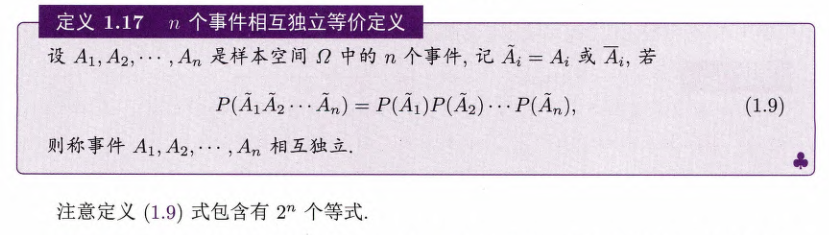小概率原理：即使事件 $A$$A$ 是小概率事件，即事件 $A$$A$ 在一次试验中不易发生，但是随着实验次数 $n$$n$ 的增加，事件 $A$$A$ 发生的概率接近于 $1$$1$.

#### 两两独立

定义1.18 $n$$n$ 个事件两两独立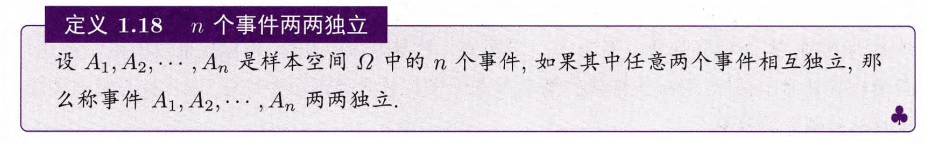相互独立的事件列一定是两两独立的，反之则未必.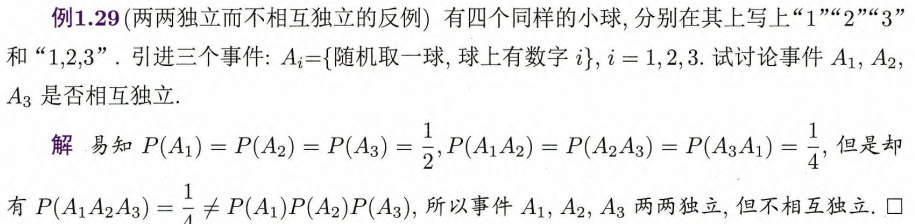定义1.19 独立事件列## 第二章 随机变量及其分布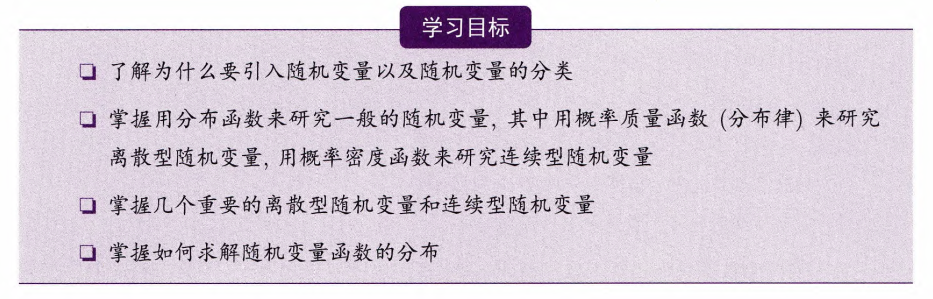### 2.1 随机变量的概念

取一个样本空间到直线 $\mathbb{R}$$\R$ 之间的映射 $X$$X$，该映射把基本事件对应于直线上的一个点，这个映射就称为随机变量（random variable, r.v.）.

• 直观上，随机变量是取值随实验结果而定且有一定概率分布的变量；

• 数学角度上的严格定义（定义2.1 随机变量）：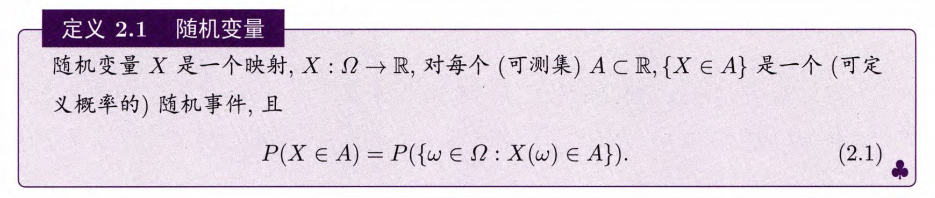通常我们用大写的英文字母 $X,Y,Z,W$$X,Y,Z,W$ 等表示随机变量，而用小写的字母 $x,y,a,b$$x,y,a,b$ 等表示实数.

随机变量取哪些值以及取这些值的概率，称为随机变量的分布（distribution）.

### 2.2 离散型随机变量的分布

离散型随机变量，就是取值为离散值的随机变量.